Next: Inductance Up: Magnetic Induction Previous: Worked Example 4: AC

## Worked Example 5: AC motors

Question: An AC motor has an internal resistance of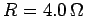. When powered by a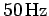AC supply of peak voltage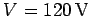it draws a peak current of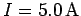. What is the peak back-emf produced by the motor? What is the peak power delivered to the motor by the AC supply? What is the peak rate of energy loss as heat in the motor? What is the peak useful power produced by the motor? What is the efficiency (i.e., the ratio of the peak useful power output to the peak power delivered) of such a motor?

Answer: Ifis the peak applied voltage, and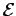the peak back-emf, then the peak applied voltage must equal the sum of the peak voltage drops across the motor, or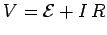. It follows that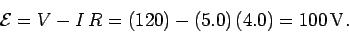The peak power delivered by the AC supply is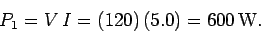Energy is lost as heat in the motor at the peak rate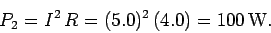The peak useful power produced by the motor is the difference between the peak power supplied to the motor and the peak power dissipated as heat: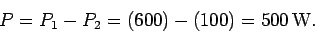The peak useful power is also given by the product of the peak back-emf and the peak current flowing through the motor [see Eq. (226)],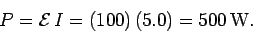The efficiency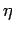is the ratio of the peak useful power output of the motor to the peak power supplied, or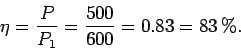Next: Inductance Up: Magnetic Induction Previous: Worked Example 4: AC
Richard Fitzpatrick 2007-07-14